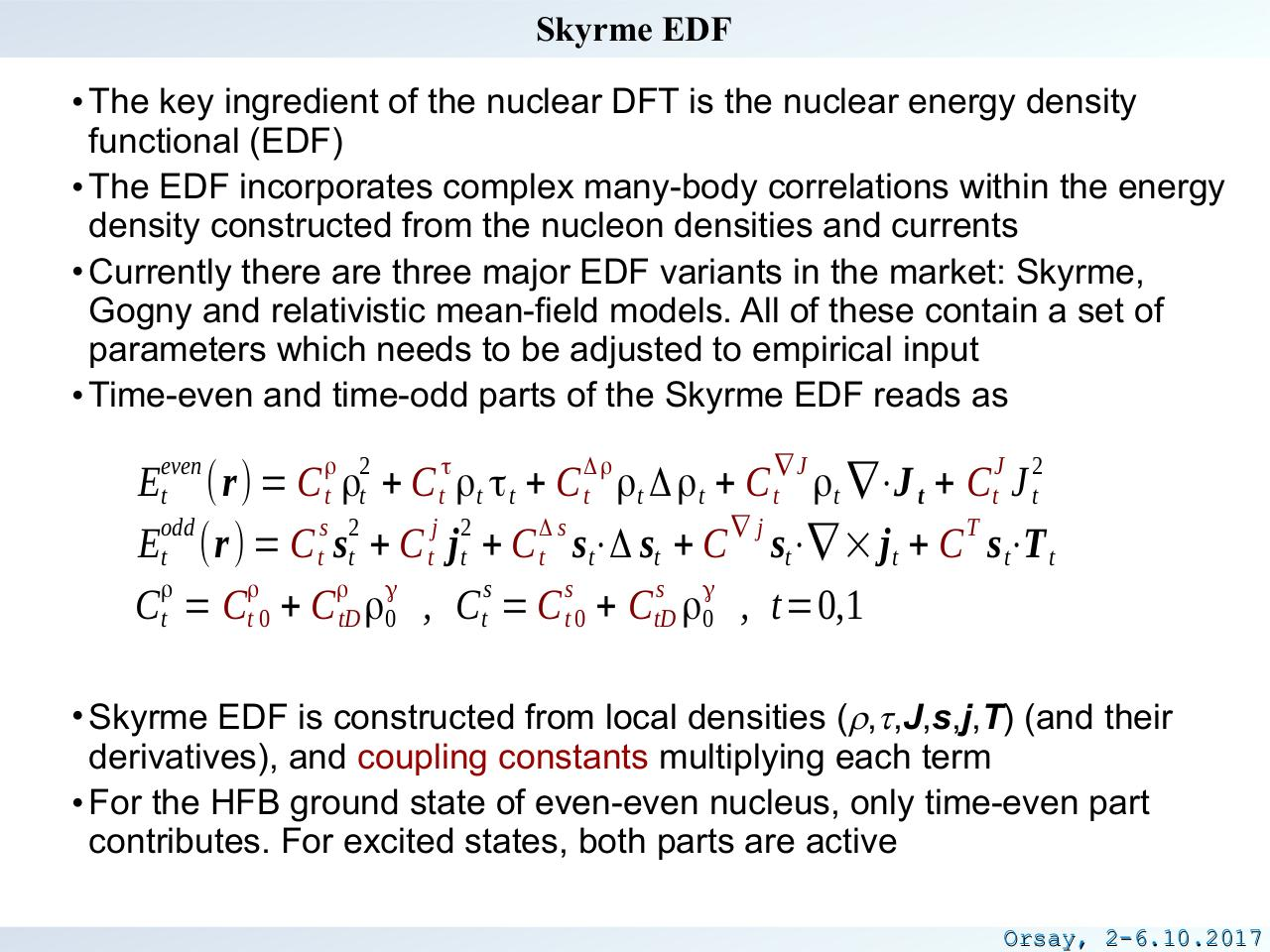# kortelainen.pdfPage 1 2 34521

#### Text preview

Skyrme EDF
• The key ingredient of the nuclear DFT is the nuclear energy density
functional (EDF)
• The EDF incorporates complex many-body correlations within the energy
density constructed from the nucleon densities and currents
• Currently there are three major EDF variants in the market: Skyrme,
Gogny and relativistic mean-field models. All of these contain a set of
parameters which needs to be adjusted to empirical input
• Time-even and time-odd parts of the Skyrme EDF reads as
even

ρ 2

τ

Δρ

∇J

J

2

Et (r) = C t ρt + C t ρt τ t + C t ρt Δ ρt + C t ρt ∇⋅J t + Ct J t
odd
s 2
j 2
Δs
∇j
T
Et (r) = C t st + C t j t + C t s t⋅Δ st + C st⋅∇ × j t + C s t⋅T t
ρ
ρ
ρ γ
s
s
s γ
Ct = Ct 0 + C tD ρ0 , Ct = C t 0 + CtD ρ0 , t=0,1
• Skyrme EDF is constructed from local densities (r,t,J,s,j,T) (and their
derivatives), and coupling constants multiplying each term
• For the HFB ground state of even-even nucleus, only time-even part
contributes. For excited states, both parts are active
Orsay, 2-6.10.2017